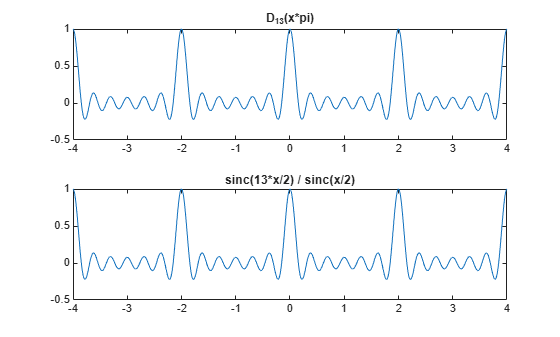# diric

Dirichlet or periodic sinc function

## Syntax

y = diric(x,n)

## Description

example

y = diric(x,n) returns the Dirichlet Function of degree n evaluated at the elements of the input array x.

## Examples

collapse all

Compute and plot the Dirichlet function between $-2\pi$ and $2\pi$ for N = 7 and N = 8. The function has a period of $2\pi$ for odd N and $4\pi$ for even N.

x = linspace(-2*pi,2*pi,301); d7 = diric(x,7); d8 = diric(x,8); subplot(2,1,1) plot(x/pi,d7) ylabel('N = 7') title('Dirichlet Function') subplot(2,1,2) plot(x/pi,d8) ylabel('N = 8') xlabel('x / \pi')The Dirichlet and sinc functions are related by ${D}_{N}\left(\pi x\right)=sinc\left(Nx/2\right)/sinc\left(x/2\right)$. Show this relationship for $N=6$. Avoid indeterminate expressions by specifying that the ratio of sinc functions is ${\left(-1\right)}^{\mathit{k}\left(\mathit{N}-1\right)}$ for $\mathit{x}=2\mathit{k}$, where $\mathit{k}$ is an integer.

xmax = 4; x = linspace(-xmax,xmax,1001)'; N = 6; yd = diric(x*pi,N); ys = sinc(N*x/2)./sinc(x/2); ys(~mod(x,2)) = (-1).^(x(~mod(x,2))/2*(N-1)); subplot(2,1,1) plot(x,yd) title('D_6(x*pi)') subplot(2,1,2) plot(x,ys) title('sinc(6*x/2) / sinc(x/2)')Repeat the calculation for $\mathit{N}=13$.

N = 13; yd = diric(x*pi,N); ys = sinc(N*x/2)./sinc(x/2); ys(~mod(x,2)) = (-1).^(x(~mod(x,2))/2*(N-1)); subplot(2,1,1) plot(x,yd) title('D_{13}(x*pi)') subplot(2,1,2) plot(x,ys) title('sinc(13*x/2) / sinc(x/2)')## Input Arguments

collapse all

Input array, specified as a real scalar, vector, matrix, or N-D array. When x is nonscalar, diric is an element-wise operation.

Data Types: double | single

Function degree, specified as a positive integer scalar.

Data Types: double | single

## Output Arguments

collapse all

Output array, returned as a real-valued scalar, vector, matrix, or N-D array of the same size as x.

collapse all

### Dirichlet Function

The Dirichlet function, or periodic sinc function, is

${D}_{N}\left(x\right)=\left\{\begin{array}{ll}\frac{\mathrm{sin}\left(Nx/2\right)}{N\mathrm{sin}\left(x/2\right)}\hfill & x\ne 2\pi k,\text{ }k=0,±1,±2,±3,...\hfill \\ {\left(-1\right)}^{k\left(N-1\right)}\hfill & x=2\pi k,\text{ }k=0,±1,±2,±3,...\hfill \end{array}$

for any nonzero integer N.

This function has period 2π for odd N and period 4π for even N. Its maximum value is 1 for all N, and its minimum value is –1 for even N. The magnitude of the function is 1/N times the magnitude of the discrete-time Fourier transform of the N-point rectangular window.

## Version History

Introduced before R2006a